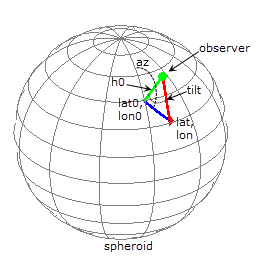# lookAtSpheroid

Line of sight intersection with oblate spheroid

## Syntax

``````[lat,lon,slantrange] = lookAtSpheroid(lat0,lon0,h0,az,tilt,spheroid)``````

## Description

example

``````[lat,lon,slantrange] = lookAtSpheroid(lat0,lon0,h0,az,tilt,spheroid)``` computes the latitude and longitude (`lat` and `lon`) of the first point of intersection of the line-of-sight from a viewpoint in space with the surface of an oblate spheroid. If the line-of-sight does not intersect with the spheroid, `lat` and `lon` contain `NaN`s.`lat0` and `lon0` are the geodetic coordinates of the viewpoint on the reference spheroid, `spheroid`. `h0` specifies the geodetic height of the viewpoint in space above the spheroid. The `az` and `tilt` arguments specify the direction of the view (line-of-sight) as the azimuth angle, measured clockwise from North, and a tilt angle. The following figure illustrates these measurements.The optional `slantrange` output argument returns the (3-D Euclidean) distance from the viewpoint to the intersection. All angles are in degrees. ```

## Examples

collapse all

Create a reference spheroid. Specify the length unit as kilometers.

`spheroid = wgs84Ellipsoid('km');`

Define location of view in space. The units for `h0` match the units of the spheroid (kilometers).

```lat0 = 0; lon0 = -100; h0 = 35786;```

Define the view from space in terms of the azimuth angle and tilt.

```az = 45; tilt = 6;```

Calculate the point on the spheroid at which the view first intersects with the spheroid. The example also returns the distance in kilometers between the viewpoint in space and the first point of intersection with the spheroid.

`[lat,lon,slantrange] = lookAtSpheroid(lat0,lon0,h0,az,tilt,spheroid)`
```lat = 25.7991 ```
```lon = -71.3039 ```
```slantrange = 3.7328e+04 ```

## Input Arguments

collapse all

Geodetic latitude of the viewpoint on the spheroid, specified as a scalar value, vector, matrix, or N-D array.

Data Types: `single` | `double`

Geodetic longitude of the viewpoint on the spheroid, specified as a scalar value, vector, matrix, or N-D array.

Data Types: `single` | `double`

Height of the viewpoint in space above the spheroid, specified as a scalar value, vector, matrix, or N-D array. `h0` must be in units that match the spheroid input.

Data Types: `single` | `double`

Azimuth angle of view from space, specified as a scalar value, vector, matrix, or N-D array. Measured in degrees, clockwise from north.

Data Types: `single` | `double`

Tilt angle of view from space, specified as scalar value, vector, matrix, or N-D array. Measured in degrees, relative to a vector pointing downward toward the nadir point. The nadir point is the point on the spheroid directly below the viewpoint, with geodetic coordinates (lat0, lon0, 0). When the tilt is zero (0), the line-of-sight is directed at the nadir point itself. `Tilt` can be in the range ```[0 180]``` but for large angles and all angles greater than or equal to 90 degrees, there is no intersection with the spheroid.

Data Types: `single` | `double`

Reference spheroid, specified as a `referenceEllipsoid`, `oblateSpheroid`, or `referenceSphere` object. Use the constructor for one of these three classes, or the `wgs84Ellipsoid` function, to construct a spheroid object. You cannot directly pass in the name of the reference spheroid. Instead, pass the name to `referenceEllipsoid` or `referenceSphere` and use the resulting object.

## Output Arguments

collapse all

Latitude of the first point of intersection with the spheroid, returned as a scalar value, vector, matrix, or N-D array. If the line-of-sight does not intersect with the spheroid, `lat` contains `NaN`s.

Longitude of the first point of intersection with the spheroid, returned as a scalar value, vector, matrix, or N-D array. If the line-of-sight does not intersect with the spheroid, `lon` contains `NaN`s.

Distance from the viewpoint to the intersection with the spheroid, returned as a scalar value, vector, matrix, or N-D array. Units match the `LengthUnit` property of the input spheroid object.

## Version History

Introduced in R2016b